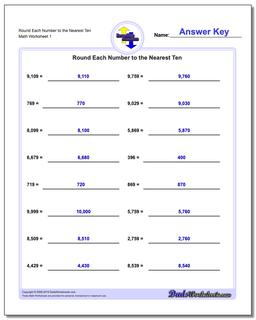# Math Worksheets: Rounding Numbers: Rounding Numbers: Round Each Number to the Nearest Ten## Round Each Number to the Nearest Ten

PropertyValue
DescriptionRound Each Number to the Nearest Ten: When the place value digit in a rounding numbers problem is a nine and you round up, it requires carrying. These rounding worksheets help practice this sometimes confusing extra step.
Resource TypeWorksheet